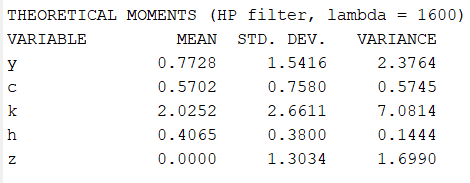# Simulated moments vs data moments

Hi.
When I run a simple RBC model,
mineRBC.mod (544 Bytes)
the moments of the model are presented as follows:For example the standard deviation of the consumption variable is equal to: 0.7580
But the standard deviation of real data for this variable is 0.04
I think the standard deviation obtained in the model is very large

This is also the case for other variables.

Does this indicate that the model is incorrect or is there a problem with my calculations for the data? I first run the Hodrick Prescott filter and then calculate the standard deviation(on the cycle).

.

Your TFP shock has a standard deviation of 100 percent, so the data is of course not comparable.
Also, 4 percent in the data for consumption is rather large. Which country are you considering?